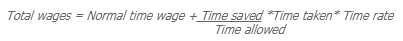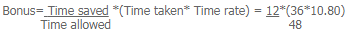Courses

# Halsey, Rowan Schemes - Employee Cost and Incentive Systems, Cost Management B Com Notes | EduRev

## B Com : Halsey, Rowan Schemes - Employee Cost and Incentive Systems, Cost Management B Com Notes | EduRev

The document Halsey, Rowan Schemes - Employee Cost and Incentive Systems, Cost Management B Com Notes | EduRev is a part of the B Com Course Cost Management.
All you need of B Com at this link: B Com

(1) Halsey scheme:

F.A. Halsey of the U.S.A. introduced this scheme.

Under this scheme, for performing a job, operation or task, a standard time is specified.

The hourly rate is fixed & the workers are guaranteed so that even if, within the standard time specified, the job is not completed by them, guaranteed time rate may be received by them. The worker becomes entitled to bonus, if he is in a position to complete the job in less than the specified time; bonus being equal to his time wage for 50% of the time saved in addition to the time wage which he is entitled for the actual time worked.

The total earning is obtained by multiplying the sum of time allowed & time taken by half the hourly rate.

Advantages: (a) The scheme & the calculation of the remuneration are easily understandable by the worker.

(b) As time wage is guaranteed, penalty is not imposed on the slow workers; whereas rewards are provided to the slow workers for their efficiency.

(c) The workers are encouraged to save as much time as possible due to the bonus, because for the higher time saved bonus will be higher.

(d) Employers are enabled to obtain more output from the workers under the scheme, & as a result of that, per unit fixed overhead get diminished.

Disadvantages: (a) Since the employers & employees share the savings in time, this may not be liked by many employees’ organizations & they argue that the workers should get the entire benefits as the savings is done by them.

(b) Compared to the other incentive plans, the workers are being offered less incentives under this scheme.

(c) Apart from the workers, savings in time also depends upon the tool’s standards, materials, and machinery & working conditions. So the desired result cannot be expected unless the best of these are assured.

(d) Chances of more spoilage, wastage, defectives & breakdown of machinery are there under this scheme, as for the purpose of maximizing the bonus, the workers will try to save as much time as possible. As a result, greater supervision cost has to be involved.

The Halsey-Weir Scheme: Under the Halsey-Weir scheme, a worker is entitled to bonus which is equal to his time wage for 331/3% (often 30%) of the time saved; instead of 50% in case of the Halsey scheme. Thus except the above, there is no difference between the Halsey scheme & the Halsey-Weir scheme.

(2) The Rowan Scheme:

David Rowan of Glasgow (U.S.A) introduced the scheme, under which time wage is guaranteed as in the case of Halsey scheme. For the performance of a job, standard time is fixed; otherwise operation or task is exactly in the same manner as in the case of Halsey scheme. For the hours of his actual work, the worker gets his time wage; on this point also it doesn’t differ from the Halsey scheme. The bonus of the worker, who is able to finish the job in less than the allowed time, is equal to his time wage for that proportion of the time taken as the saved time bears to the time allowed. In other words, the ratio between the bonus & the time saved is equal to the ratio between the time taken & the time allowed.Or Bonus hours: Time Saved :: Time Taken: Time Allowed

(a) Because the premium is proportionate to the time saved, if the rate has been wrongly fixed, the effect will be less serious. So Rowan scheme is safer than the Halsey scheme, as far as the point of view of employer is concerned.

(b) The worker is in the most advantageous position when 50% of the time allowed is saved by him, because otherwise his earning per hour will increase at a diminishing rate, if any more time is saved by him. As a result, the chances of wastage, defectives, breakdown etc. will be less as there is a limit to speed.

(c) Fixed overhead per unit will be lower as a result of higher output.

(d) Since both the employer & the employee enjoys the time saved; though proportion is not the same as in the case of the Halsey scheme, to some extent labour cost also diminishes.

(e) Better wage is earned by the employees; their improvement in efficiency is rewarded.

(a) The workers do not like the idea of sharing the savings by both employer & employee, since the time is saved by the workers.

(b) The bonus hours will not exceed the 25% of the time allowed in any case.

(c) Apart from workers efficiency, saving of time depends upon standard of tools, materials & implements & also upon the working conditions. No useful purpose will be served unless the best of these are assured.

Example: Calculate the total earnings of a worker & the effectively rate of labour wages per hour where payment of bonus is under

(a) the Halsey (50%) scheme &
(b) the Rowan scheme from the below mentioned particulars:

Basic wage rate per hour – \$.10.80, Time allowed for the job – 48 hours, Actual time taken – 36 hours.

Solution: (a) Under Halsey (50%) Scheme:

Total wages = Normal time wage + 50% of (time saved*time rate)

Normal time wage = 36 hours @ \$.10.80 =  \$. 388.80

Bonus = 50% of (Time saved*time rate) = 50% (12*10.80)  \$. 64.80

Total Wages  =  453.60

Effective hourly rate = \$.453.60/36 = \$.12.60

Alternatively;

Total wages = (Time taken+50% of time saved)*time rate

= (36 hours+50% of 12)*\$.10.80 = \$.453.60

(b) Under Rowan Scheme:Normal time wage = 36 hours @ \$. 10.80 = 388.80= 97.20

Effective hourly rate = \$.486.00/36 = \$. 13.50

Alternatively;

Bonus as a percentage of Time rate = (Time saved/Time allowed)*100 = (12/48)*100 = 25%

Bonus = 25% of \$.10.80 = \$. 2.70

Bonus as a fraction of time rate = (Time saved/Time allowed)*Time rate = (12/48)*10.80 = \$.2.70

Effective hourly rate for time taken = \$.10.80+\$.2.70 = \$.13.50

Total Wages = 36 hours*\$.13.50 = \$. 486

Example: 40 hours is taken by a worker to do job for which time allowed is 50 hours. \$. 1.25 per hour is his daily rate. Calculate the works cost of the job under the following methods of payment of wages: (i) Time rate; (ii) Piece rate; (iii) Halsey plan & (iv) Rowan plan.
Additional information: (i) Material cost \$.60; (ii) Factory overhead 125% of wages

Solution: Calculation of wages under different methods:

(i)Time Rate: Wages for 40 hours (actual time taken) @ \$. 1.25 = 50.00

(ii)Piece Rate: Wages for 50 hours (time allowed for the job) @ \$. 1.25  = 62.50

(iii) Halsey Plan: Normal time wage = 40 hours @ \$.1.25  = 50.00

Bonus = 50% of (Time saved*Time rate) =50% of (10*1.25) =   6.25

(iv) Rowan Plan: Normal time wage = 40 hours @ \$.1.25  = 50.00

Bonus = Time saved/Time allowed*(Time taken*Time rate) = 10/50*(40*1.25) = 10.00

Total = 60.00

Offer running on EduRev: Apply code STAYHOME200 to get INR 200 off on our premium plan EduRev Infinity!

## Cost Management

52 videos|51 docs

,

,

,

,

,

,

,

,

,

,

,

,

,

,

,

,

,

,

,

,

,

,

,

,

,

,

,

;